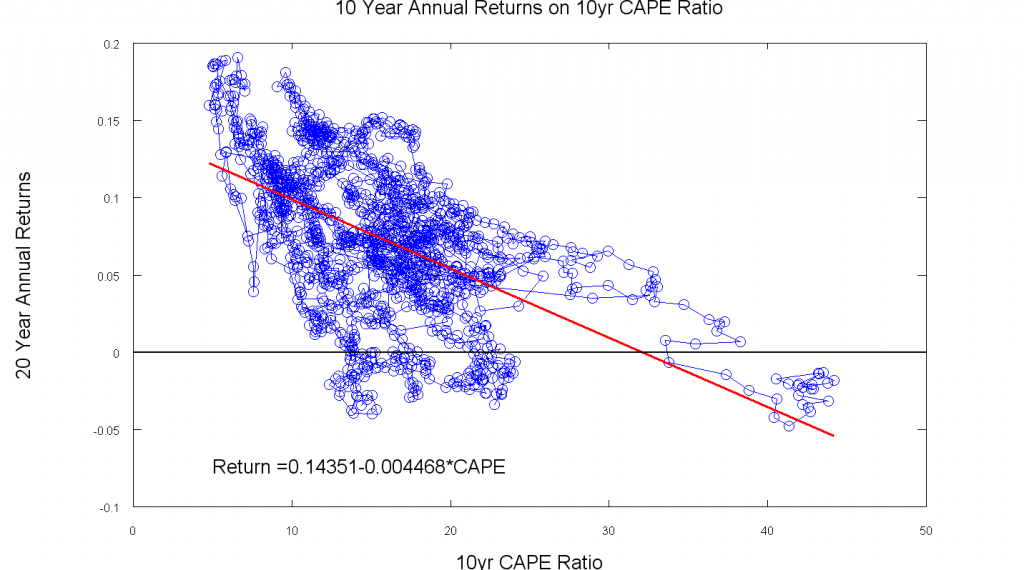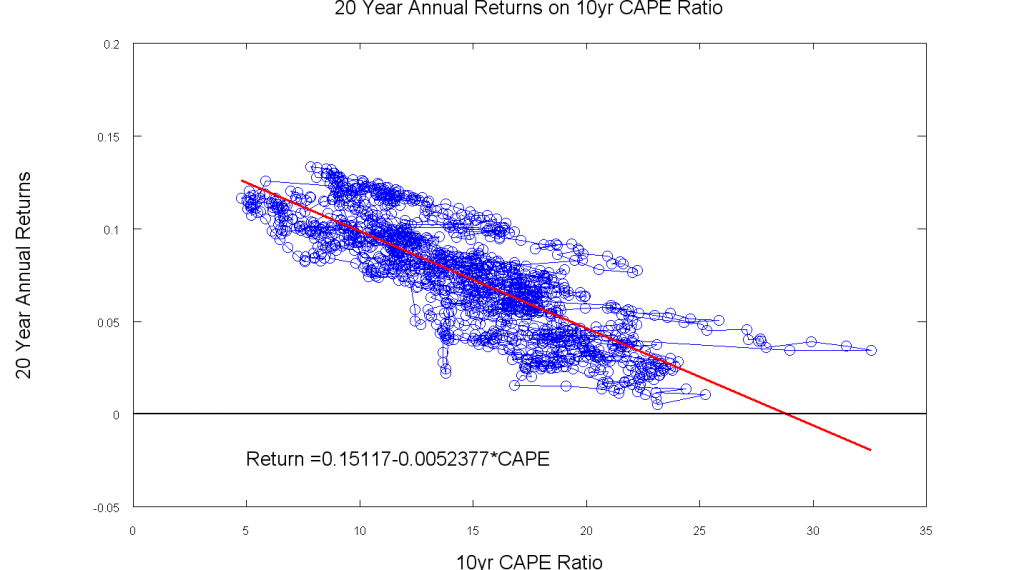# Market Valuation

Dec 302010

What market return should we expect over the next 10 to 20 years?

Reviewing the Campbell-Shiller Data

What return should you expect to get on your stock market investments over the next 10 to 20 years?  According to data compiled by Yale University’s Robert Shiller, the average annual real return (i.e. after adjusting for the effects of inflation) on an S&P500-like portfolio over the past 139 years has been 8.2%.  The geometric average annual real return, which is a better indication of the real return realized by a buy and hold investor, was 6.6%.  You might think that these numbers would provide a reasonable estimate of future market returns.  However, all stock market eras are not created equal.  In fact, if we look at the cyclically-adjusted price-earnings (CAPE) ratio and the subsequent 10 or 20 year market return, we can see that times of high market valuation (high CAPE ratio) have been followed by periods of low market returns, and periods of low market valuation (low CAPE ratio) have been followed by periods of high returns.  This relationship is clear in the graphs below.The Octave code used to generate these plots is available here.

There is some reasonable logic supporting this valuation-return relationship.  When the CAPE ratio is low, the dividend yield of the stock market tends to be high.  This means that investors are getting a significant portion of their return from the cash flow generated by the current earnings of their investments, and they require a relatively small capital gain (price rise) to realize a high total return.  In contrast, when the CAPE ratio is high, dividend yields have tended to be low, so investors are getting little cash flow from the current earnings of their investments, and they are instead relying on capital gains for the bulk of their returns.  So, returns have tended to be high when valuations are well supported by cash flow, and they have tended to be low when investors are relying primarily on capital gains.

There has been a lot of discussion about the statistical significance of the regression relationships shown in these graphs.  The statistical relationship is much weaker than it first appears because most of the 10 and 20 year periods analyzed are overlapping, so we are effectively using same data multiple times in the regression analysis.  There are only about 6 independent 20-year periods and 12 independent 10-year periods in the data.  Nevertheless, the logic behind the valuation-return relationship is compelling, and similar valuation-return relationships have been shown in international stock markets.   I think this is a reasonable relationship to consider when estimating likely levels of future market returns.

I suspect (time will tell) that the 20-year regression line will flatten somewhat as we get enough data to include the 20-year returns from the very high CAPEs of the late nineties.  There is already some indication of this flattening (or bending) as the most extreme CAPEs are above the current regression line.

Where are we now?

The variation in the CAPE over time is shown here.Note that although valuation has tended to eventually revert to the mean, the process has been quite slow, and the market has at times stayed above or below the average valuation level for a decade or more.  For this reason, using the CAPE ratio to time short term moves in and out of the market is unlikely to be a profitable strategy.

At present, the CAPE of about 22.5 is about 37% above its long term historical average of 16.4.  The real return implied by the two regression equations is:

10-year estimate:

Return = 0.1435 – 0.004468*CAPE =  0.1435 – 0.004468*22.5 = 0.043 = 4.3%

20-year estimate:

Return = 0.1512 – 0.005238*CAPE =  0.1512 – 0.005238*22.5 = 0.033 = 3.3%

The two regression equations give estimates in the range of 3.3% to 4.3% real return.  If we assume an inflation rate in the 2-3% range, this leads to a nominal return estimate in the range of 5.3% to 7.3%.  This estimate applies to the arithmetic return, so the geometric estimate would be slightly lower. This estimated range is below the historical average, but it is much better than the S&P500 returns of the past 10 years.

Conclusions

Niels Bohr (maybe) once said “Predictions are very hard, especially about the future”.  This is especially true for forecasts about future stock market returns.  Obviously, there is no perfect method for forecasting future returns, and even the best methods are only rough estimates.  Smart investors plan for an uncertain world.  However, regressions using the Campbell-Shiller data are a logical way to generate a rough estimate of future market returns.  The estimated returns provide a reasonable starting point when planning for the future, and the analysis helps to temper any tendency towards excessive optimism or pessimism at times of extreme market valuation.

Note:  This review of the Campbell-Shiller data is inspired by the regressions posted by Brad Delong on his blog.  I’ve updated the regressions with the latest data, added some info to the graphs, and posted the code I used to run the regressions and generate the plots, but return equations and plots are very similar to what he posted in 2009.